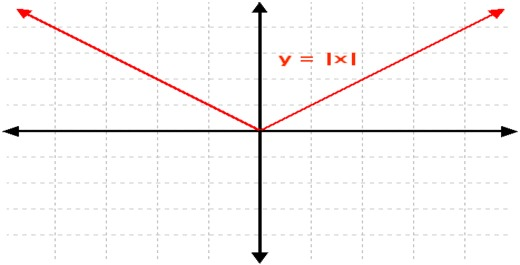Our Address: FZ LLC G 37 & G 34, Block 13, Al Sufouh Road Knowledge Park, Dubai PO Box : 501703

# Absolutely Important | Absolute ValueSo, what exactly do we mean by Absolute Value/ Modulus? Lamely speaking, whatever comes out of the two parallel bars |y| => will always be non-negative (it may be 0 also).

Eg. |-7|= 7, likewise, |7| = 7.

Let us say we have |y|, where y is any random number on the number line. Then, |y| means it is the distance of y from the origin! Can we have a negative distance? Not really! Thus, the absolute value is always non-negative. It is always positive, the only different case is |0|= 0.

Now, if we have |x|> 5, this inequality means the distance of x from origin is greater than 5. Now, this distance, may be on the left side of 0, or the right side of 0. Left side means, x will lie farther (leftwards) than -5 and right side means, x will lie farther (rightwards) than 5. Thus, x < -5 or x > 5.

Typical Important properties of Absolute Value:

1. x/|x| may have only two values, 1 and -1, provided .

If x > 0, then x/|x| = 1

If x < 0, then x/|x| = -1

Likewise, also for |x|/x = 1 or -1

2. If x and y have the same signs, (+,+) or (-,-), the distance, of (x+y) from origin is same as the sum of the distance of x from origin and the distance of y from origin.

Eg. |3 + 5| = |3|+|5|= 8

|(-3)+(-5)|= |-3|+|-5|= 3+5=8

If x and y have opposite signs, (+, -) or (-, +), ue to the opposite signs either x or y will cancel out some value of the other, thus, the distance between x from origin and the distance between y from origin is always greater than distance of (x+y) from origin.

Eg. |5+(-3)|= |2|= 2 < (|5|+|-3|) i.e 8

Likewise, |-5+3|=|-2|=2 < (|-5|+|3|) i.e 8

3. If x and y have same signs, (+,+) or (-,-), provided

If x and y have opposite signs (+,-) or (-, +) and if same signs but, then,

More about the unique properties of Absolute Values in the next GMAT article.Till then these are enough to chew upon to get a more vivid sense of absolute values.